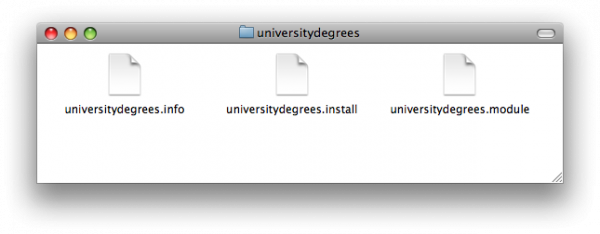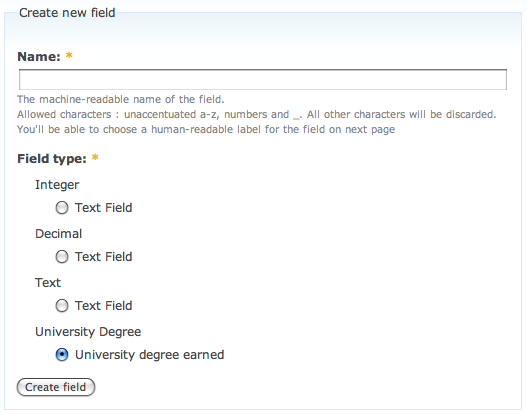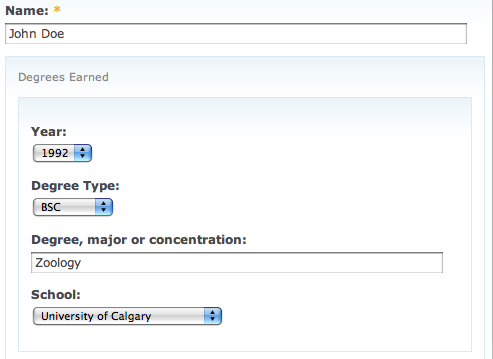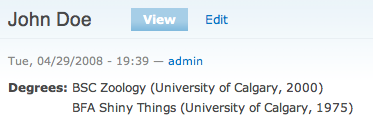# Creating a custom compound field for CCK

To start with, I just created a new folder to hold the module, and called it "universitydegrees". Into this folder, I copied the .install and .info files from another module and customized them to suit my needs (the universitydegrees.install file doesn't actually do anything, and the universitydegrees.info file is just for listing the module in the Drupal admin page). If you want to follow along (or use the files as a starting point to make something else) the files for my lame alpha version of the module are available here.The file where all the fun stuff happens is universitydegrees.module

The first method, universitydegrees_field_info(), defines how the field shows up in the CCK admin interface.

function universitydegrees_field_info() {
return array(
'universitydegrees' => array('label' => 'University Degree'),
);
}

This method works with the universitydegrees_widget_info() method, and will list any available widgets under the "University Degree" field type. In this case, I just added a simple widget description, like this:

function universitydegrees_widget_info() {
return array(
'universitydegrees_text' => array(
'label' => 'University degree earned',
'field types' => array('universitydegrees'),
),
);
}

So, a new field called "University Degree" will be available, like this:Before actually adding the field to any content type, we need to define what data it's going to store. This happens in the universitydegrees_field_settings() method:

function universitydegrees_field_settings($op,$field) {
switch ($op) { case 'save': return array('year', 'degreetype', 'programme', 'institution'); case 'database columns':$columns = array(
'year' => array('type' => 'int', 'not null' => TRUE, 'default' => '2008', 'sortable' => TRUE),
'degreetype' => array('type' => 'varchar', 'length' => 255, 'not null' => TRUE, 'default' => "''", 'sortable' => TRUE),
'programme' => array('type' => 'varchar', 'length' => 255, 'not null' => TRUE, 'default' => "''", 'sortable' => TRUE),
'institution' => array('type' => 'varchar', 'length' => 255, 'not null' => TRUE, 'default' => "''", 'sortable' => TRUE),
);
return $columns; case 'filters': return array( 'default' => array( 'list' => '_universitydegrees_filter_handler', 'list-type' => 'list', 'operator' => 'views_handler_operator_or', 'value-type' => 'array', 'extra' => array('field' =>$field),
),
);
}
}

The 'save' portion defines what values will be saved. The 'database columns' portion defines the MySQL code to generate the fields in the database to store each of the values. In this case, 'year' will be an int and the remaining values will be varchar(255) text strings.

Now, to define how the widget that is used to edit the values should behave. This is the universitydegrees_widget() method.

function universitydegrees_widget($op, &$node, $field, &$items) {
$options_year = _universitydegrees_values_year();$options_degreetype = _universitydegrees_values_degreetype();
$options_institution = _universitydegrees_values_institution(); switch ($op) {

case 'form':
$form = array();$form[$field['field_name']] = array('#tree' => TRUE); if ($field['multiple']) {
$form[$field['field_name']]['#type'] = 'fieldset';
$form[$field['field_name']]['#description'] = t('Degrees Earned');
$delta = 0; foreach (range($delta, $delta + 2) as$delta) {
$item =$items[$delta];$form[$field['field_name']][$delta]['#type'] = 'fieldset';
$form[$field['field_name']][$delta]['year'] = array( '#type' => 'select', '#title' => t('Year'), '#default_value' => array_search($items[$delta]['year'],$options_year),
'#options' => $options_year, );$form[$field['field_name']][$delta]['degreetype'] = array(
'#type' => 'select',
'#title' => t('Degree Type'),
'#default_value' => array_search($items[$delta]['degreetype'], $options_degreetype), '#options' =>$options_degreetype,
'#required' => ($delta == 0) ?$field['required'] : FALSE,
);
$form[$field['field_name']][$delta]['programme'] = array( '#type' => 'textfield', '#title' => t('Degree, major or concentration'), '#default_value' =>$items[$delta]['programme'], '#required' => ($delta == 0) ? $field['required'] : FALSE, );$form[$field['field_name']][$delta]['institution'] = array(
'#type' => 'select',
'#title' => t('School'),
'#default_value' => array_search($items[$delta]['institution'], $options_institution), '#options' =>$options_institution,
'#required' => ($delta == 0) ?$field['required'] : FALSE,
);
}
}
else {
$form[$field['field_name']]['#type'] = 'fieldset';
$form[$field['field_name']]['year'] = array(
'#type' => 'select',
'#title' => t('Year'),
'#default_value' => array_search($items['year'],$options_year),
'#options' => $options_year, '#required' =>$field['required'],
);
$form[$field['field_name']][$delta]['degreetype'] = array( '#type' => 'select', '#title' => t('Degree Type'), '#default_value' => array_search($items['degreetype'], $options_degreetype), '#options' =>$options_degreetype,
'#required' => $field['required'], );$form[$field['field_name']]['programme'] = array( '#type' => 'select', '#title' => t('Degree, major or concentration'), '#default_value' =>$items['programme'],
'#required' => $field['required'], );$form[$field['field_name']]['institution'] = array( '#type' => 'select', '#title' => t('School'), '#default_value' => array_search($items['institution'], $options_institution), '#options' =>$options_institution,
'#required' => $field['required'], ); } return$form;

case 'process form values':

foreach ($items as$delta => $item) { // don't store empty stuff. if (empty($items[$delta]['year']) &&$delta > 0 ) {
unset($items[$delta]);
} else {
// do an array lookup to store the actual value of the selection, not just the number of its index position.
$items[$delta]['year'] = $options_year[$items[$delta]['year']];$items[$delta]['degreetype'] =$options_degreetype[$items[$delta]['degreetype']];
$items[$delta]['institution'] = $options_institution[$items[$delta]['institution']]; } } } } The $options_year, $options_degreetype, and $options_institution variables are just calling methods that return arrays of values. I did this initially to separate the options from the various places they are used so I could change where the data comes from more easily (it'd be really cool to tie these into taxonomies or something more user-editable...)

The 'form' portion generates the portion of the form that is used to edit the content associated with an instance of the field. It has two sub-portions, one for handling multiple values, and one for single values. I'm really not sure why that isn't collapsed into a single chunk that can grok both single and multiple values, but I was following a recipe and left it that way. For now... The only portion I really cared about was the multivalue stuff anyway, because that's how I'll be using the content type.

The code for that portion defines a fieldset, called "Degrees Earned" and starts pumping out chunks of forms to present editors for each value. It creates a nested fieldset for each value, and presents a Select field for "Year", "Degree Type" and "Institution" - and a textfield for "Degree, major or concentration".

One thing that I didn't like in the out-of-the-box widget behaviour was that it stored the index of the value, rather than the actual value itself, in the database. While that worked, it wasn't ideal - if I changed the list of options, the index values would be invalid. So I modified the code to lookup the actual value selected and store that for the select widgets. (the #default_value portions of the code present the current value, if any, while editing).

The 'process form values' portion does any processing of the values prior to saving in the database. This is where I convert the stored values from plain, dumb, indexes to a more meaningful text string containing the actual selection.

Here's what the form looks like, with the widgets in place:Now that we have a field defined, have provided a way to save values in the database, and described how the form widgets should behave, we need to display values on the node page. I took a shortcut and only defined a single hard-coded way to present the values. I'll eventually work in a way to customize the display using a list of formatters. In the meantime, the universitydegrees_field_formatter() method handles the conversion from raw data into displayable text.

function universitydegrees_field_formatter($field,$item, $formatter,$node) {
$text = '';$text = $item['degreetype'] . ' ' .$item['programme'] . ' (' . $item['institution'] . ', ' .$item['year'] . ')';
return \$text;
}

With the formatter in place, a node with this CCK field type will look like this:And that's it. Now, I can add a University Degree to any CCK content type, and be able to define all four values that describe a degree awarded to an individual. This pattern could easily be generalized for other compound data types, as well.

Next, I need to expose full Views functionality, so each value can be used for filtering and sorting Views. And, I need to provide a more flexible way to display the values, using the formatters. And, I need to abstract the lists of Years, Degrees and Institutions so that they are editable by users without having to modify the source code for the module...